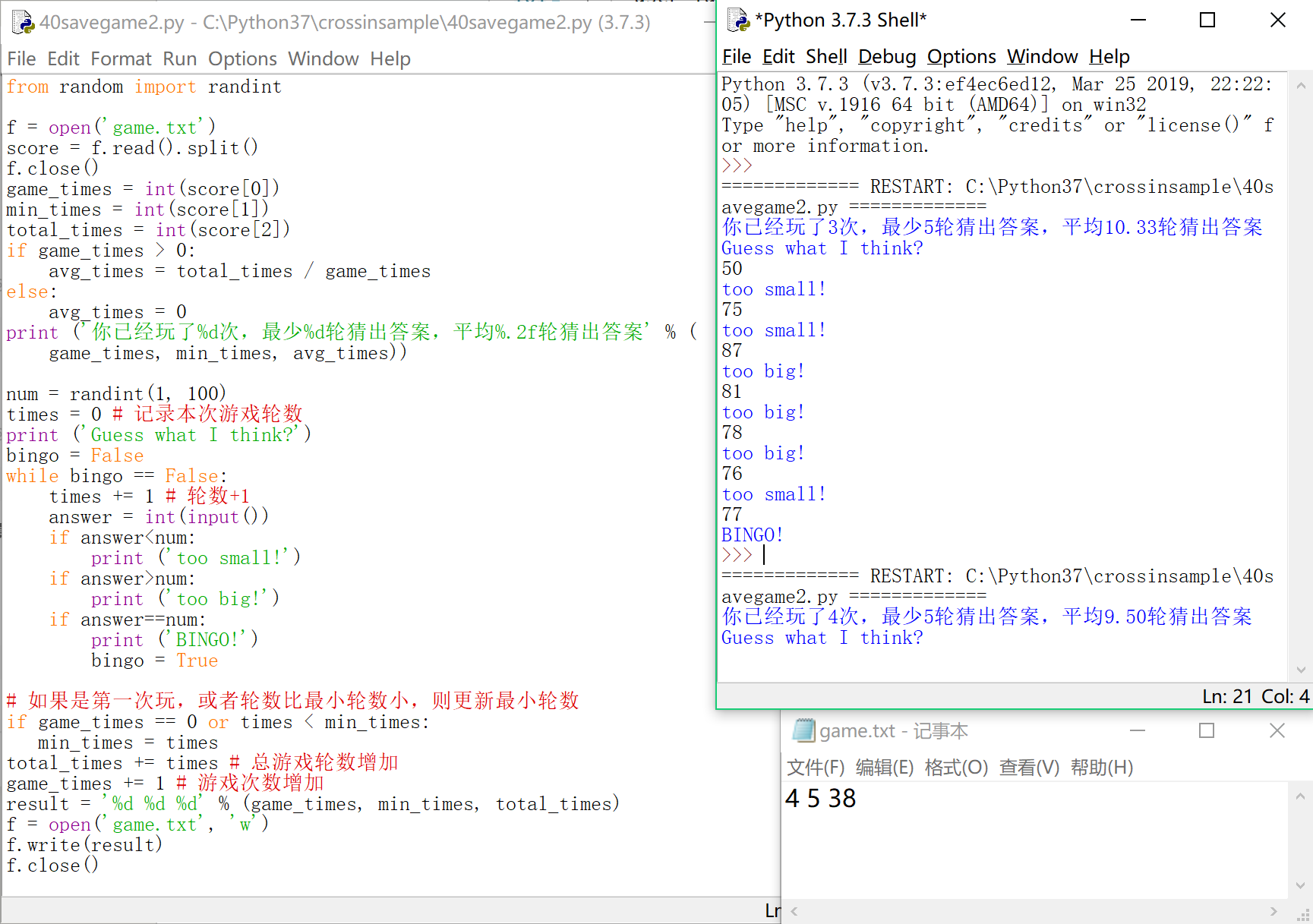## 【Python 第40课】用文件保存游戏（2）

``times = 0``

``times += 1``

``````if game_times == 0 or times < min_times:
min_times = times``````

``total_times += times``

``game_times += 1``

``result = '%d %d %d' % (game_times, min_times, total_times)``

``````f = open('game.txt', 'w')
f.write(result)
f.close()``````